Related Articles

# Split Pandas Dataframe by column value

• Last Updated : 20 Aug, 2020

Sometimes in order to analyze the Dataframe more accurately, we need to split it into 2 or more parts. The Pandas provide the feature to split Dataframe according to column index, row index, and column values, etc.

Let’ see how to Split Pandas Dataframe by column value in Python?

Now, let’s create a Dataframe:

## Python3

 `# importing pandas library``import` `pandas as pd`` ` `# Initializing the nested list with Data-set``player_list ``=` `[[``'M.S.Dhoni'``, ``36``, ``75``, ``5428000``],``               ``[``'A.B.D Villers'``, ``38``, ``74``, ``3428000``],``               ``[``'V.Kholi'``, ``31``, ``70``, ``8428000``],``               ``[``'S.Smith'``, ``34``, ``80``, ``4428000``],``               ``[``'C.Gayle'``, ``40``, ``100``, ``4528000``],``               ``[``'J.Root'``, ``33``, ``72``, ``7028000``],``               ``[``'K.Peterson'``, ``42``, ``85``, ``2528000``]]`` ` `# creating a pandas dataframe``df ``=` `pd.DataFrame(player_list, ``                  ``columns ``=` `[``'Name'``, ``'Age'``, ``                             ``'Weight'``, ``'Salary'``])`` ` `# show the dataframe``df`

Output: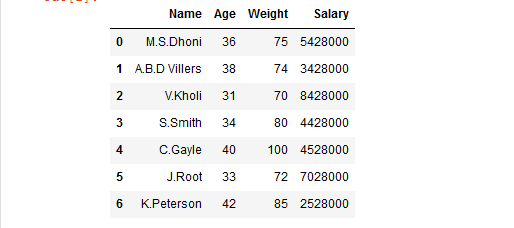Method 1: Using boolean masking approach.

This method is used to print only that part of dataframe in which we pass a boolean value True.

Example 1:

## Python3

 `# importing pandas library``import` `pandas as pd`` ` `# Initializing the nested list with Data-set``player_list ``=` `[[``'M.S.Dhoni'``, ``36``, ``75``, ``5428000``],``               ``[``'A.B.D Villers'``, ``38``, ``74``, ``3428000``],``               ``[``'V.Kholi'``, ``31``, ``70``, ``8428000``],``               ``[``'S.Smith'``, ``34``, ``80``, ``4428000``],``               ``[``'C.Gayle'``, ``40``, ``100``, ``4528000``],``               ``[``'J.Root'``, ``33``, ``72``, ``7028000``],``               ``[``'K.Peterson'``, ``42``, ``85``, ``2528000``]]`` ` `# creating a pandas dataframe``df ``=` `pd.DataFrame(player_list, ``                  ``columns ``=` `[``'Name'``, ``'Age'``, ``                             ``'Weight'``, ``'Salary'``])`` ` `# splitting the dataframe into 2 parts``# on basis of 'Age' column values``# using Relational operator``df1 ``=` `df[df[``'Age'``] >``=` `37``]`` ` `# printing df1``df1`

Output: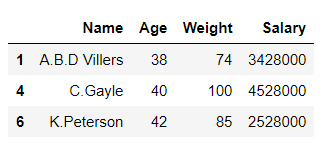## Python3

 `df2 ``=` `df[df[``'Age'``] < ``37``]`` ` `# printing df2``df2`

Output: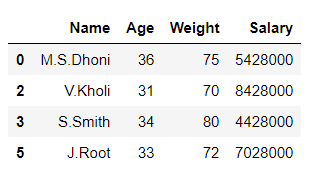In the above example, the data frame ‘df’ is split into 2 parts ‘df1’ and ‘df2’ on the basis of values of column ‘Age‘.

Example 2:

## Python3

 `# importing pandas library``import` `pandas as pd`` ` `# Initializing the nested list with Data-set``player_list ``=` `[[``'M.S.Dhoni'``, ``36``, ``75``, ``5428000``],``               ``[``'A.B.D Villers'``, ``38``, ``74``, ``3428000``],``               ``[``'V.Kholi'``, ``31``, ``70``, ``8428000``],``               ``[``'S.Smith'``, ``34``, ``80``, ``4428000``],``               ``[``'C.Gayle'``, ``40``, ``100``, ``4528000``],``               ``[``'J.Root'``, ``33``, ``72``, ``7028000``],``               ``[``'K.Peterson'``, ``42``, ``85``, ``2528000``]]`` ` `# creating a pandas dataframe``df ``=` `pd.DataFrame(player_list, ``                  ``columns ``=` `[``'Name'``, ``'Age'``, ``                             ``'Weight'``, ``'Salary'``])`` ` `# splitting the dataframe into 2 parts``# on basis of 'Weight' column values``mask ``=` `df[``'Weight'``] >``=` `80`` ` `df1 ``=` `df[mask]`` ` `# invert the boolean values``df2 ``=` `df[~mask]`` ` `# printing df1``df1`

Output: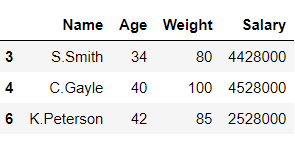## Python3

 `# printing df2``df2`

Output: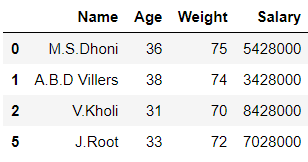In the above example, the data frame ‘df’ is split into 2 parts ‘df1’ and ‘df2’ on the basis of values of column ‘Weight‘.

Method 2: Using Dataframe.groupby().

This method is used to split the data into groups based on some criteria.

Example:

## Python3

 `# importing pandas library``import` `pandas as pd`` ` `# Initializing the nested list with Data-set``player_list ``=` `[[``'M.S.Dhoni'``, ``36``, ``75``, ``5428000``],``               ``[``'A.B.D Villers'``, ``38``, ``74``, ``3428000``],``               ``[``'V.Kholi'``, ``31``, ``70``, ``8428000``],``               ``[``'S.Smith'``, ``34``, ``80``, ``4428000``],``               ``[``'C.Gayle'``, ``40``, ``100``, ``4528000``],``               ``[``'J.Root'``, ``33``, ``72``, ``7028000``],``               ``[``'K.Peterson'``, ``42``, ``85``, ``2528000``]]`` ` `# creating a pandas dataframe``df ``=` `pd.DataFrame(player_list, ``                  ``columns ``=` `[``'Name'``, ``'Age'``, ``                             ``'Weight'``, ``'Salary'``])`` ` `# splitting the dataframe into 2 parts``# on basis of 'Salary' column values``# using dataframe.groupby() function``df1, df2 ``=` `[x ``for` `_, x ``in` `df.groupby(df[``'Salary'``] < ``4528000``)]`` ` `# printing df1``df1`

Output: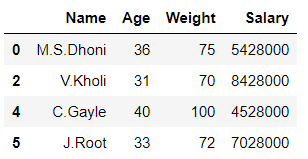## Python3

 `# printing df2``df2`

Output: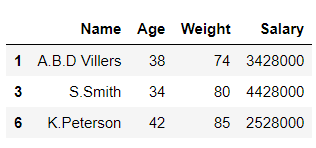In the above example, the data frame ‘df’ is split into 2 parts ‘df1’ and ‘df2’ on the basis of values of column ‘Salary‘.

Attention geek! Strengthen your foundations with the Python Programming Foundation Course and learn the basics.

To begin with, your interview preparations Enhance your Data Structures concepts with the Python DS Course. And to begin with your Machine Learning Journey, join the Machine Learning – Basic Level Course

My Personal Notes arrow_drop_up